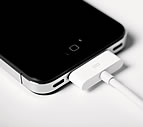×

# cube

3 ENTRIES FOUND:
1 cube /ˈkjuːb/ noun
plural cubes
1 cube
/ˈkjuːb/
noun
plural cubes
Learner's definition of CUBE
[count]
: an object that has six square sides
see picture at geometry
mathematics : the number that results from multiplying a number by itself twice
compare 1square 3
US, informal + humorous :
2 cube /ˈkjuːb/ verb
cubes; cubed; cubing
2 cube
/ˈkjuːb/
verb
cubes; cubed; cubing
Learner's definition of CUBE
[+ object]
: to cut (food) into small cubes
mathematics : to multiply (a number) by itself twice usually used as cubed
compare 3square 2